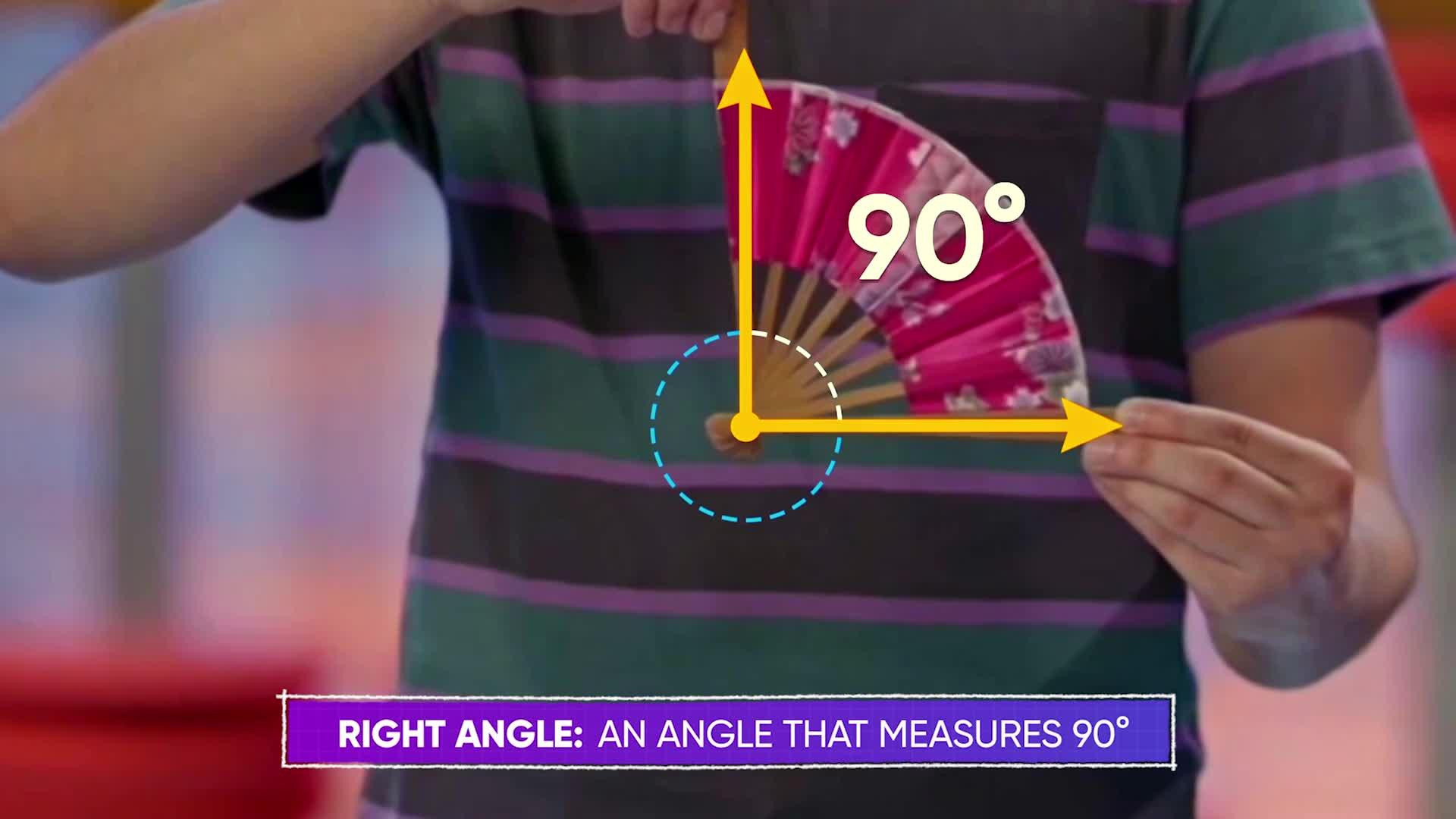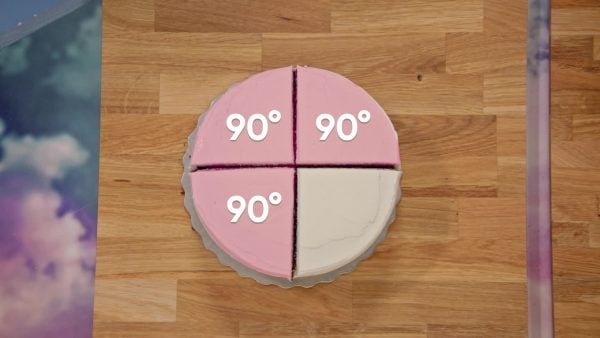Angles Math Video for Kids - 3rd, 4th, & 5th Grades
1%
It was processed successfully!WHAT IS AN ANGLE?

An angle is the rotational space between two straight lines (rays) that share a common endpoint (vertex). You can measure and draw angles using a protractor.

To better understand angles…

WHAT IS AN ANGLE?. An angle is the rotational space between two straight lines (rays) that share a common endpoint (vertex). You can measure and draw angles using a protractor. To better understand angles…

## LET’S BREAK IT DOWN!

### SkateboardingMarco and Alexandria are at a skate park. They watch some of the more experienced skateboarders doing tricks and name the tricks based on angles. An angle measures rotation. Angles are measured in degrees (°), and there are 360° in a circle. 1° is [ggfrac]1/360[/ggfrac] of a circle. A skateboarder does a trick, rotating one-half circle. What angle do they rotate through? Imagine a ray on the front of their skateboard; a second ray moves with the skateboard's front as the skateboard rotates. A one-half turn covers half the degrees in a circle, and 360 divided by 2 is 180. Therefore, Marco calls the trick a 180°. Another skateboarder does a trick where she rotates one-fourth of a full circle. 360 divided by 4 is 90, so Alexandria calls the trick a 90°. You have seen 90° angles in a lot of shapes. A 90° angle has a special name: a right angle. The angles in a square or rectangle are right angles. Angles larger than right angles but smaller than 180° are called obtuse, and angles smaller than right angles are called acute. Try this yourself: What is the name of a trick where a skateboarder rotates three-quarters of a circle?

Skateboarding Marco and Alexandria are at a skate park. They watch some of the more experienced skateboarders doing tricks and name the tricks based on angles. An angle measures rotation. Angles are measured in degrees (°), and there are 360° in a circle. 1° is [ggfrac]1/360[/ggfrac] of a circle. A skateboarder does a trick, rotating one-half circle. What angle do they rotate through? Imagine a ray on the front of their skateboard; a second ray moves with the skateboard's front as the skateboard rotates. A one-half turn covers half the degrees in a circle, and 360 divided by 2 is 180. Therefore, Marco calls the trick a 180°. Another skateboarder does a trick where she rotates one-fourth of a full circle. 360 divided by 4 is 90, so Alexandria calls the trick a 90°. You have seen 90° angles in a lot of shapes. A 90° angle has a special name: a right angle. The angles in a square or rectangle are right angles. Angles larger than right angles but smaller than 180° are called obtuse, and angles smaller than right angles are called acute. Try this yourself: What is the name of a trick where a skateboarder rotates three-quarters of a circle?

### Pops and LocksMarcos is learning new dance moves. To accomplish a move, Marcos needs to hold out his leg at a 45° angle and his arm at 135° from his body. You can use a protractor to measure or draw a specific angle. To measure an angle, place the protractor with its hole over the vertex of the angle. Put the 0° line along one ray of the angle. Measure the angle by following the rotation on the protractor and counting the degrees up from 0°. When you get to the second ray, there are two sets of numbers marked on the protractor, but only one of them has counted up from 0°. That is the angle measurement. You can draw an angle using a protractor by drawing the first ray and placing the protractor on the vertex and along that ray. Count from 0° along the protractor until you get to the angle measurement you want to draw. Put a tick mark or dot on your paper at this spot. Then you can use a ruler to connect the vertex to your tick mark. Viola! You have made an angle. Try this yourself: Draw an angle, and then use a protractor to measure the angle.

Pops and Locks Marcos is learning new dance moves. To accomplish a move, Marcos needs to hold out his leg at a 45° angle and his arm at 135° from his body. You can use a protractor to measure or draw a specific angle. To measure an angle, place the protractor with its hole over the vertex of the angle. Put the 0° line along one ray of the angle. Measure the angle by following the rotation on the protractor and counting the degrees up from 0°. When you get to the second ray, there are two sets of numbers marked on the protractor, but only one of them has counted up from 0°. That is the angle measurement. You can draw an angle using a protractor by drawing the first ray and placing the protractor on the vertex and along that ray. Count from 0° along the protractor until you get to the angle measurement you want to draw. Put a tick mark or dot on your paper at this spot. Then you can use a ruler to connect the vertex to your tick mark. Viola! You have made an angle. Try this yourself: Draw an angle, and then use a protractor to measure the angle.

### Making Angles with CakeAdesina ordered three circle cakes and had the bakers divide the cakes into pieces with different-sized angles. April and Marcos are allowed to take 2 pieces of cake each, but only if they find the total angle measurement of the cake they are taking. However, the baker forgot to label some of the angles! One cake is divided into 4 equal sections. Since the whole circle cake is 360°, one-fourth of the cake is 90°. You can add angles exactly like you add other numbers or measurements. 90° + 90° + 90° + 90° = 360°. Another cake is divided into five pieces: 90°, 90°, 90°, 60°, and one unlabeled piece. Since four 90° angles add up to a full 360°, 60° combined with the missing angle must equal 90°. 90 - 60 = 30, so the missing angle is 30°. The last cake is divided into four pieces: 90°, 90°, 125°, and the one unlabeled piece. Since 90 + 90 = 180, 125° combined with the unlabeled piece must make 180°. 180 – 125 = 55. The last piece is 55°. Marcos picks two slices of cake to eat: one marked with 55° and another with 30°. His slices total 55° + 30° = 85°. April chooses two different pieces 60° and 125°. Her slices total 60° + 125° = 185°. Try this yourself: A circular cake is cut into four pieces, measuring 100°, 50°, 90°, and one unknown measurement. What is the missing angle measurement?

Making Angles with Cake Adesina ordered three circle cakes and had the bakers divide the cakes into pieces with different-sized angles. April and Marcos are allowed to take 2 pieces of cake each, but only if they find the total angle measurement of the cake they are taking. However, the baker forgot to label some of the angles! One cake is divided into 4 equal sections. Since the whole circle cake is 360°, one-fourth of the cake is 90°. You can add angles exactly like you add other numbers or measurements. 90° + 90° + 90° + 90° = 360°. Another cake is divided into five pieces: 90°, 90°, 90°, 60°, and one unlabeled piece. Since four 90° angles add up to a full 360°, 60° combined with the missing angle must equal 90°. 90 - 60 = 30, so the missing angle is 30°. The last cake is divided into four pieces: 90°, 90°, 125°, and the one unlabeled piece. Since 90 + 90 = 180, 125° combined with the unlabeled piece must make 180°. 180 – 125 = 55. The last piece is 55°. Marcos picks two slices of cake to eat: one marked with 55° and another with 30°. His slices total 55° + 30° = 85°. April chooses two different pieces 60° and 125°. Her slices total 60° + 125° = 185°. Try this yourself: A circular cake is cut into four pieces, measuring 100°, 50°, 90°, and one unknown measurement. What is the missing angle measurement?

## INTRO TO ANGLES VOCABULARY

Angle
The rotational space between two rays connected at a common point.
Degree
A unit of measurement for angles. A full circle measures 360°, so 1° is [ggfrac]1/360[/ggfrac] of a circle.
Right angle
An angle that measures 90°, or one-quarter turn on a circle.
Acute angle
An angle that measures less than 90°.
Obtuse angle
An angle that measures more than 90° and less than 180°.
Protractor
A measurement tool used to measure the angle between two lines (rays).
The point where two lines (rays) meet and form an angle.

## INTRO TO ANGLES DISCUSSION QUESTIONS

### What is a degree?

A degree is a unit of measure for angles. It is equal to [ggfrac]1/360[/ggfrac]th of a circle.

### What is different between an acute angle, an obtuse angle, and a right angle?

An acute angle is less than 90°, an obtuse angle is between 90° and 180°, and a right angle is equal to 90°.

### Two-dimensional (2D) shapes can be sorted based on their angle measurements. What kind of angles are in a rectangle?

A rectangle has all 90° angles, which means it has all right angles.

### When you measure an angle using a protractor, how do you know what set of numbers to use?

First, I need to make sure that the vertex of the angle is under the hole in the protractor. Next, I need to line one of the straight lines up with the 0° line on the protractor. Then I need to trace along the protractor from 0° until I reach the second ray. The set of numbers that I have counted up from 0° tell me the measure of an angle.

### If you change the orientation of two rays, does the size of the angle change?

No, an angle's orientation does not affect its size.
X

## Success

We’ve sent you an email with instructions how to reset your password.
Ok

## Choose Your Free Trial Period### 7 DaysContinue to Lessons### 90 DaysGet 90 days free by inviting other teachers to try it too.

Share with Teachers

## Get 90 Days Free

### By inviting 4 other teachers to try it too.

4 required

Skip, I will use a 7 days free trial

## Thank You!

Enjoy your free 90 days trial